Important Questions (1 Mark): Polynomials

# Important Questions (1 Mark): Polynomials

Test Description

## 25 Questions MCQ Test Mathematics (Maths) Class 10 | Important Questions (1 Mark): Polynomials

Important Questions (1 Mark): Polynomials for Class 10 2023 is part of Mathematics (Maths) Class 10 preparation. The Important Questions (1 Mark): Polynomials questions and answers have been prepared according to the Class 10 exam syllabus.The Important Questions (1 Mark): Polynomials MCQs are made for Class 10 2023 Exam. Find important definitions, questions, notes, meanings, examples, exercises, MCQs and online tests for Important Questions (1 Mark): Polynomials below.
Solutions of Important Questions (1 Mark): Polynomials questions in English are available as part of our Mathematics (Maths) Class 10 for Class 10 & Important Questions (1 Mark): Polynomials solutions in Hindi for Mathematics (Maths) Class 10 course. Download more important topics, notes, lectures and mock test series for Class 10 Exam by signing up for free. Attempt Important Questions (1 Mark): Polynomials | 25 questions in 25 minutes | Mock test for Class 10 preparation | Free important questions MCQ to study Mathematics (Maths) Class 10 for Class 10 Exam | Download free PDF with solutions
 1 Crore+ students have signed up on EduRev. Have you?
Important Questions (1 Mark): Polynomials - Question 1

### Form a quadratic polynomial, sum of whose zeroes is 3 and product of whose zeroes is 2 solution

Detailed Solution for Important Questions (1 Mark): Polynomials - Question 1

Sum of zeros = 3/1
-b/a = 3/1
Product of zeros = 2/1
c/a = 2/1
This gives
a = 1
b = -3
c = -2,
ax2+bx+c
So,  x2-3x+2

Important Questions (1 Mark): Polynomials - Question 2

### If x + 2 is a factor of x3 – 2ax2 + 16, then value of a is

Detailed Solution for Important Questions (1 Mark): Polynomials - Question 2

Given ,g(x)=x+2=0
x=-2

P(x)=x3-2ax2+16
p(-2)=(-23)-2×a×(-22)+16
= -8 - 8a +16
= 8 - 8a
⇒ -8a = -8
⇒ a=1
Hence, value of a=1

So option B is correct answer.

Important Questions (1 Mark): Polynomials - Question 3

### If 3 + 5 – 8 = 0, then the value of (3)3 + (5)3 – (8)3 is

Detailed Solution for Important Questions (1 Mark): Polynomials - Question 3

Given :
3 + 5 + (-8) = 0.

Then,
3³ + 5³ + (-8)³ = ?

Using, identity...
If a+b+c=0, then a³ + b ³ + c³ = 3abc

Let a = 3, b = 5 and c = -8.

Here, a + b + c = 3 + 5 + (-8) = 0.

Then, a³ + b³ + c³ = 3³ + 5³ + (-8)³
= 3× 3 × 5 × -8
= 9 × -40
= -360

Important Questions (1 Mark): Polynomials - Question 4

If one of the factors of x2 + x – 20 is (x + 5), then other factor is

Detailed Solution for Important Questions (1 Mark): Polynomials - Question 4

Using mid-term splitting,
x2+x-20=x2+5x-4x-20=x(x+5)-4(x+5)
Taking common x+5
(x+5)(x-4) , so the other factor is x-4

Important Questions (1 Mark): Polynomials - Question 5

If α,β be the zeros of the quadratic polynomial 2x2 + 5x + 1, then value of α + β + αβ =

Detailed Solution for Important Questions (1 Mark): Polynomials - Question 5

P(x) = 2x² + 5x + 1
Sum of roots = -5/2
Product of roots = 1/2
Therefore substituting these values,
α + β +αβ
=(α + β) + αβ
= -5/2 + 1/2
= -4/2
= -2

Important Questions (1 Mark): Polynomials - Question 6

If α,β be the zeros of the quadratic polynomial 2 – 3x – x2, then α + β =

Detailed Solution for Important Questions (1 Mark): Polynomials - Question 6

If α  and β are the zeros of the polynomial then
(x−α)(x−β) are the factors of the polynomial
Thus, (x−α)(x−β) is the polynomial.
So, the polynomial =x− αx − βx + αβ
=x− (α + β)x + αβ....(i)
2 − 3x − x2 = x2 + 3x − 2....(ii)

Now, comparing equation (i) and (ii),we get,
−(α + β) = 3
α + β = −3

Important Questions (1 Mark): Polynomials - Question 7

Quadratic polynomial having sum of it's zeros 5 and product of it's zeros – 14 is –

Detailed Solution for Important Questions (1 Mark): Polynomials - Question 7

The quadratic equation is of the form  x- (sum of zeros) x + (product of zeros)
=x- 5x - 14

Important Questions (1 Mark): Polynomials - Question 8

If x = 2 and x = 3 are zeros of the quadratic polynomial x2 + ax + b, the values of a and b respectively are :

Detailed Solution for Important Questions (1 Mark): Polynomials - Question 8

Zeros of the polynomials are the values which gives zero when their value is substituted in the polynomial
When x=2,
x2+ax+b =(2)2+a*2+b=0
4+2a+b=0
b=-4-2a    ….1
When x=3,
(3)2+ 3a + b=0
9 + 3a + b=0
Substituting
9 + 3a - 4 - 2a =0
5 + a =0
a = -5
b = 6

Important Questions (1 Mark): Polynomials - Question 9

If 3 is a zero of the polynomial f(x) = x4 – x3 – 8x2 + kx + 12, then the value of k is –

Detailed Solution for Important Questions (1 Mark): Polynomials - Question 9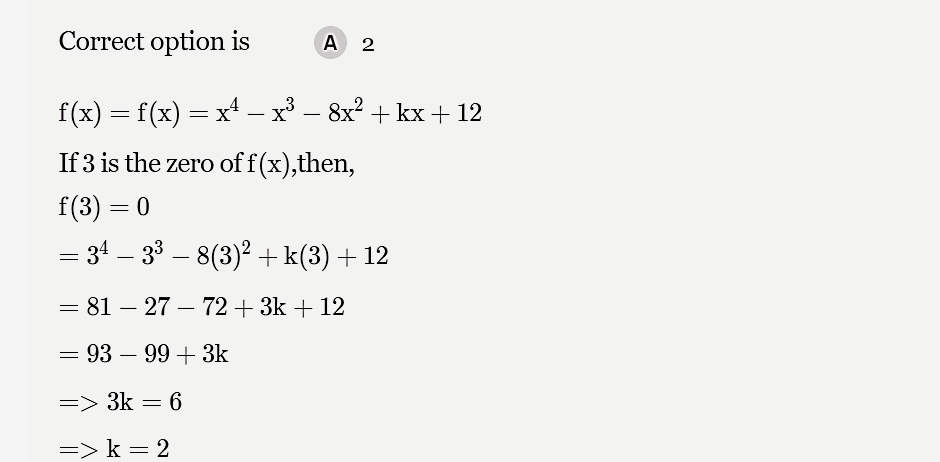Important Questions (1 Mark): Polynomials - Question 10

The sum and product of zeros of the quadratic polynomial are – 5 and 3 respectively the quadratic polynomial is equal to –

Important Questions (1 Mark): Polynomials - Question 11

On dividing x3 – 3x2 + x + 2 by polynomial g(x), the quotient and remainder were x – 2 and 4 – 2x respectively then g(x) :

Detailed Solution for Important Questions (1 Mark): Polynomials - Question 11

We have remainder theorem as
x3-3x2+x+2=q(x)*g(x)+r(x)
x3-3x2+x+2=(x-2)g(x)+(4-2x)
g(x)=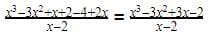So by division method,
g(x)= x- x + 1

Important Questions (1 Mark): Polynomials - Question 12

If the polynomial 3x2 – x3 – 3x + 5 is divided by another polynomial x – 1 – x2, the remainder comes out to be 3, then quotient polynomial is –

Detailed Solution for Important Questions (1 Mark): Polynomials - Question 12

Let's assume the quotient polynomial as ax + b.

Now, (x - 1 - x^2)(ax + b) + 3 = 3x^2 - x^3 - 3x + 5

ax^3 + bx^2 + ax^2 - ax^2 - bx - a + 3 = -x^3 + 3x^2 - 3x + 5

ax^3 + (b - 1)x^2 + (a - b)x + (3 - a) = -x^3 + 3x^2 - 3x + 5

Equating the coefficients of the same degree terms on both sides, we get:

For x^3: a = -1 For x^2: b - 1 = 3 => b = 4 For x: a - b = -3 => a - 4 = -3 => a = 1 For constant: 3 - a = 5 => a = -2

Therefore, the quotient polynomial is x + 4.

Hence, the quotient polynomial is x + 4.

Important Questions (1 Mark): Polynomials - Question 13

If x + 2 is a factor of x3 – 2ax2 + 16, then value of a is

Important Questions (1 Mark): Polynomials - Question 14

If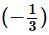is the zero of the cubic polynomial f(x) = 3x3 – 5x2 – 11x – 3 the other zeros are :

Important Questions (1 Mark): Polynomials - Question 15

If p and q are the zeroes of the polynomial x2- 5x - k. Such that p - q = 1, find the value of K

Important Questions (1 Mark): Polynomials - Question 16

Let p(x) = ax2 + bx + c be a quadratic polynomial. It can have at most –

Important Questions (1 Mark): Polynomials - Question 17

The graph of the quadratic polynomial ax2 + bx + c, a ≠ 0 is always –

Detailed Solution for Important Questions (1 Mark): Polynomials - Question 17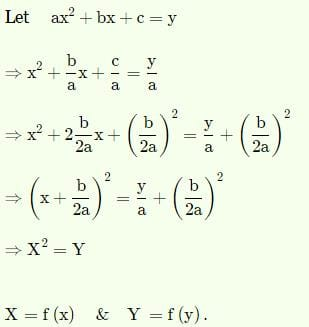So it s an equation of a parabola.

The graph will be that of a parabola.

Important Questions (1 Mark): Polynomials - Question 18

If 2 and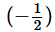as the sum and product of its zeros respectively then the quadratic polynomial f(x) is –

Important Questions (1 Mark): Polynomials - Question 19

If α and β are the zeros of the polynomial f(x) = 16x2 + 4x – 5 then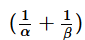is equal to –

Important Questions (1 Mark): Polynomials - Question 20

If α and β are the zeros of the polynomial f(x) = 15x2 – 5x + 6 then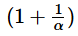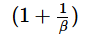is equal to –

Detailed Solution for Important Questions (1 Mark): Polynomials - Question 20

(1+1/α) (1+1/β)

= (α+1/α) (β+1/β)

=αβ + α + β + 1 / αβ

=( 2/5 + 1/3+ 1)  /  2/5

=26/15 X 5/2 = 13/3

Important Questions (1 Mark): Polynomials - Question 21

If the sum of the two zeros of x3 + px2 +qx + r is zero, then pq =

Detailed Solution for Important Questions (1 Mark): Polynomials - Question 21

Sum of two solutions is zero ,so both are inverse of each other. So we have the solutions as a,-a,b.
(x-a)(x+a)(x+b)=(x2-a2)(x+b)=x3+bx2-a2x-a2b
pq=-a2b=r

Important Questions (1 Mark): Polynomials - Question 22

Let a = 0 and p(x) be a polynomial of degree greater than 2. If p(x) leaves remainders a and – a when divided respectively by x + a and x – a, the remainder when p(x) is divided by x2 – ais

Detailed Solution for Important Questions (1 Mark): Polynomials - Question 22

Let P(x)=(x−a)(x+a)Q(x)+rx+s
When we divide by x+a, we get
P(−a)=(−a−a)(−a+a)Q(−a)+r(−a)+s=a
⇒s−ra=a   ...(1)
And when we divide by x−a, we get
P(a)=(a−a)(a+a)Q(a)+ra+s=−a
⇒s+ra=−a    ...(2)
Solving (1) and (2), we get
s=0,r=−1
Hence, P(x)=(x−a)(x+a)Q(x)−x
And when we divide by x2-a2, it leaves a remainder −x.

Important Questions (1 Mark): Polynomials - Question 23

If one root of the polynomial x2 + px + q is square of the other root, then –

Detailed Solution for Important Questions (1 Mark): Polynomials - Question 23

Let one root be a, other root is a2.
(x-a)(x-a2)=x2+(-a2-a)x+a3
p3=(-a2-a)3=-a6-3a5-3a4-a3
-q(3p-1)= -a3(3(-a2-a)-1)=3a5+3a4+a3
q2=a6
Substituting the values
-a6-3a5-3a4-a3+3a5+3a4+a3+a6=0

Important Questions (1 Mark): Polynomials - Question 24

If α,β are the zeros of x2 + px + 1 and γ,δ be those of x2 + qx + 1, then the value of (α–γ) (β–γ) (α+δ) (β+δ) =

Detailed Solution for Important Questions (1 Mark): Polynomials - Question 24

Alpha(a) and beta(b) are roots of x^2 + px + 1

This implies that sum of roots= a+b = -p/1=-p

And the product of roots = ab = 1/1=1

Similarly ,

Gamma(c) and delta(d) are roots of x^2 + qx + 1

So c+d=-q and cd =1.

The above results can be obtained once we know that any quadratic equation has two roots and hence can be written as (x-p)(x-q)=0 where a and b are the roots .

So x^2 -(p+q)x + pq =0

Comparing this with the general form of quadratic equation :ax^2 + bx + c= 0 we get

Sum of the roots =p+q= -b/a

And product of the roots = pq = c/a}

RHS=(a-c)(b-c)(a+d)(b+d)

=(c^2-ac-bc +ab)(d^2 +bd +ad + ab)

We know ab=1

So RHS= (c^2-ac-bc +1)(d^2 +bd +ad + 1)

= (c^2)(d^2) +(a+b)c^2(d) + c^2 -(d^2)c(a+b) -(a+b)^2(cd) -(a+b)c + d^2 + bd + ad + 1

= 1 + ac+bc + c^2 - da-db - (a^2 + b^2 + 2(1)) -ac -bc + bd + ad +1

Cancelling off all the common terms,

We get c^2 +d^2-a^2-b^2

= c^2+d^2-a^2-b^2
=c^2 -a^2 + d^2 -b^2 + 2(1) -2(1){ ab=cd =1}
=c^2 + d^2 + 2cd - a^2 - b^2 - 2ab
LHS=(c+d)^2-(a+b)^2
Therefore,
But we know -p= a+b and -q = c+d
LHS= q^2-p^2

Important Questions (1 Mark): Polynomials - Question 25

The quadratic polynomial whose zeros are twice the zeros of 2x2 – 5x + 2 = 0 is –

Detailed Solution for Important Questions (1 Mark): Polynomials - Question 25

Let α and β be the roots of the given equation.
Then, α + β = 5/2 and αβ = 2/2 = 1
∴ 2α + 2β
∴ 2(α + β)
∴ 5,(2α)(2β) = 4
So, the requiared equation is :
x2−5x+4=0

## Mathematics (Maths) Class 10

25 videos|370 docs|138 tests
Information about Important Questions (1 Mark): Polynomials Page
In this test you can find the Exam questions for Important Questions (1 Mark): Polynomials solved & explained in the simplest way possible. Besides giving Questions and answers for Important Questions (1 Mark): Polynomials, EduRev gives you an ample number of Online tests for practice

## Mathematics (Maths) Class 10

25 videos|370 docs|138 tests(Scan QR code)